JEE  >  BITSAT Maths Test

# BITSAT Maths Test - JEE

Test Description

## 45 Questions MCQ Test BITSAT Mock Tests Series & Past Year Papers - BITSAT Maths Test

BITSAT Maths Test for JEE 2023 is part of BITSAT Mock Tests Series & Past Year Papers preparation. The BITSAT Maths Test questions and answers have been prepared according to the JEE exam syllabus.The BITSAT Maths Test MCQs are made for JEE 2023 Exam. Find important definitions, questions, notes, meanings, examples, exercises, MCQs and online tests for BITSAT Maths Test below.
Solutions of BITSAT Maths Test questions in English are available as part of our BITSAT Mock Tests Series & Past Year Papers for JEE & BITSAT Maths Test solutions in Hindi for BITSAT Mock Tests Series & Past Year Papers course. Download more important topics, notes, lectures and mock test series for JEE Exam by signing up for free. Attempt BITSAT Maths Test | 45 questions in 55 minutes | Mock test for JEE preparation | Free important questions MCQ to study BITSAT Mock Tests Series & Past Year Papers for JEE Exam | Download free PDF with solutions
 1 Crore+ students have signed up on EduRev. Have you?
BITSAT Maths Test - Question 1

### The area of the figure bounded by the curves y = cos x and y = sin x and the ordinates x = 0 and x = π/4 is

BITSAT Maths Test - Question 2

### The sum of the coefficients in the expansion of (1+x-3x2)3148 is

BITSAT Maths Test - Question 3

### The equation of circle which passes through the points (3,-2) and (-2,0) and whose centre lies on the line 2x-y-3=0 is

BITSAT Maths Test - Question 4

The equation of a circle which is ortho gonal to circles x2+y2+3x-5y+6=0 and 4x2+4y2-28x+29=0 and whose centre lies on the line 3x+4y+1=0 is

BITSAT Maths Test - Question 5

The least positive integral value of n for which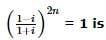BITSAT Maths Test - Question 6

The differential equation for the family of curves x2 + y2 - 2ay = 0, where a is an arbitrary constant is

BITSAT Maths Test - Question 7

The solution of differential equation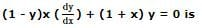Detailed Solution for BITSAT Maths Test - Question 7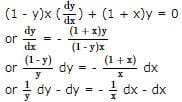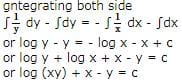BITSAT Maths Test - Question 8

Differential of sin⁻1[(1-x)/(1+x)] w.r. to √x is equal to

BITSAT Maths Test - Question 9

(d/dx)[tan⁻1((sinx+cosx)/(cosx-sinx))]

BITSAT Maths Test - Question 10

1/2! - 1/3! + 1/4! - 1/5! + ....... equals

BITSAT Maths Test - Question 11

From the top of a building of height h metres, the angle of depression of an object on the ground is α. The distance (in metres) of the object from the foot of the building is

BITSAT Maths Test - Question 12

The area of the region bounded by the curves y = |x-2|, x = 1, x = 3 and the x-axis is

BITSAT Maths Test - Question 13

The product of the perpendicular, drawn from any point on a hyperbola to its asymptotes is

BITSAT Maths Test - Question 14

The solution of the equation x(dy/dx)=y-xtan(y/x) is

BITSAT Maths Test - Question 15

The differential equation (d2y/dx2)2/3 = (y + (dy/dx))1/2 is of

BITSAT Maths Test - Question 16

The sum of first 50 terms of the series cot⁻1 3 + cot⁻1 7 + cot⁻1 13 + cot⁻1 21 + ... is

BITSAT Maths Test - Question 17

The function f(x) = cot⁻1x + x increases in the interval

BITSAT Maths Test - Question 18

(1/1)+(1/(1+2))+(1/(1+2+3))+...up to (n+1) terms is equal to

BITSAT Maths Test - Question 19

If B is a non-singular matrix and A is a square matrix, then det (B⁻1 AB) is equal to

BITSAT Maths Test - Question 20

If in a square matrix A=[aij], we find that
aij = aji ∀ i,j, then A is a

BITSAT Maths Test - Question 21

If the function f(x) = 2x3 - 9ax2 + 12a2x + 1, where a > 0, attains its max. and min. at p and q respectively such that p2 = q then a equals

BITSAT Maths Test - Question 22

The complex numbers 1,-1, i √3 from a triangle which is

BITSAT Maths Test - Question 23

If the normal at the point t on a parabola y = 4ax meet it again at t1, then t1 =

BITSAT Maths Test - Question 24

The locus of the point of intersection of two normals to the parabola x2=8y, which are at right angles to each other,is

BITSAT Maths Test - Question 25

Each line represented by equation (x₁y-xy₁)2=a2(x2+y2) is at a distance from (x₁,y₁)

BITSAT Maths Test - Question 26

The number of words formed from the letters of the word INDEPENDENCE when vowels are together is

BITSAT Maths Test - Question 27

A problem in mathematics is given to 3 students whose chances of solving individually are 1/2, 1/3, and 1/4. The probability that the problem will be solved atleast by one is

BITSAT Maths Test - Question 28

The numbers of all words formed from the letters of the word CALCUTTA is

Detailed Solution for BITSAT Maths Test - Question 28

No of letter in the given word is 8 No of repeated word is "C" 2 times, "A" 2 times and "T" 2 times. So the equation is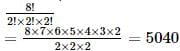BITSAT Maths Test - Question 29

The mean and variance of a Binomial distribution are 6 and 4. The parameter n is

BITSAT Maths Test - Question 30

In a ΔABC , A : B : C = 3 : 5 : 4 . Then a + b + c √2 is equal to

Detailed Solution for BITSAT Maths Test - Question 30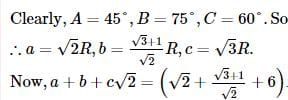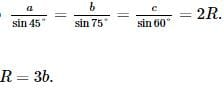BITSAT Maths Test - Question 31

If the product of the roots of the equation mx2 + 6x + 2m - 1 = 0 is -1, then the value of m is

BITSAT Maths Test - Question 32

If the sum of n terms of the series 23 + 43 + 63 + ... ∞ is 3528, then n equals

BITSAT Maths Test - Question 33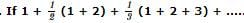to n terms is S, then S is equal to

Detailed Solution for BITSAT Maths Test - Question 33to n terms is S, then S is equal to n(n + 3)/4

BITSAT Maths Test - Question 34

If f (x) = (1)/(1 - x), then f[f{f (x)}] =

BITSAT Maths Test - Question 35

4 [cot⁻13 + cosec⁻1 (√5)] is equal to

BITSAT Maths Test - Question 36

If the parametric equation of a curve is given by x=et cos t, y=et sin t, then tangent to the curve at the point t=(π/4) makes the angle with the axis of x

BITSAT Maths Test - Question 37

The equidistance point from lines 4x+3y+10=0, 5x-12y+26=0 and 7x+24y-50=0 is

BITSAT Maths Test - Question 38

The entercepts of the plane 2x-3y+4z=12 on the co-ordinate axes are given by

BITSAT Maths Test - Question 39

The plane which passes through the point (3, 2, 0) and the line (x - 3)/1 = (y - 6)/5 = (z - 4)/4 is

BITSAT Maths Test - Question 40

The general solution of the equation tan2θ.tanθ=1 for n∈I is, θ is equal to

BITSAT Maths Test - Question 41

In a triangle A B C , s − a Δ = 1 8 , s − b Δ = 1 12 , s − c Δ = 1 24 , then b =

BITSAT Maths Test - Question 42

If tan (cotx) = cot(tanx), then sin2x is equal to

BITSAT Maths Test - Question 43

If x tan 45o cos 60o= sin 60o cot 60o, then x is equal to

BITSAT Maths Test - Question 44

If a is any vector then |axi|2+|axj|2+|axk|2=

BITSAT Maths Test - Question 45

If a+b+c=0, |a|=3, |b|=5, |c|=7, then the angle between a and b is

## BITSAT Mock Tests Series & Past Year Papers

2 videos|14 docs|143 tests
Information about BITSAT Maths Test Page
In this test you can find the Exam questions for BITSAT Maths Test solved & explained in the simplest way possible. Besides giving Questions and answers for BITSAT Maths Test, EduRev gives you an ample number of Online tests for practice

## BITSAT Mock Tests Series & Past Year Papers

2 videos|14 docs|143 tests(Scan QR code)